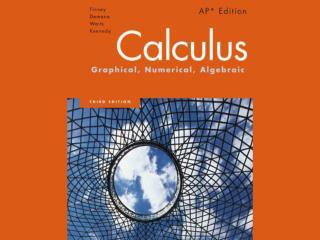DownloadDownload PresentationChapter 2

Chapter 2

Télécharger la présentationChapter 2

- - - - - - - - - - - - - - - - - - - - - - - - - - - E N D - - - - - - - - - - - - - - - - - - - - - - - - - - -
Presentation Transcript

1. Chapter 2 Limits and Continuity

2. 2.1 Rates of Change and Limits

3. Quick Review

4. Quick Review

5. Quick Review Solutions

6. Quick Review Solutions

7. What you’ll learn about • Average and Instantaneous Speed • Definition of Limit • Properties of Limits • One-Sided and Two-Sided Limits • Sandwich Theorem …and why Limits can be used to describe continuity, the derivative and the integral: the ideas giving the foundation of calculus.

8. Average and Instantaneous Speed

9. Definition of Limit

10. Definition of Limit continued

11. Definition of Limit continued

12. Properties ofLimits

13. Properties ofLimits continued Product Rule: Constant Multiple Rule:

14. Properties ofLimits continued

15. Example Properties ofLimits

16. Polynomial and Rational Functions

17. Example Limits

18. Evaluating Limits As with polynomials, limits of many familiar functions can be found by substitution at points where they are defined. This includes trigonometric functions, exponential and logarithmic functions, and composites of these functions.

19. Example Limits

20. Example Limits [-6,6] by [-10,10]

21. One-Sided and Two-Sided Limits

22. One-Sided and Two-Sided Limits continued

23. Example One-Sided and Two-Sided Limits Find the following limits from the given graph. 4 o 3 1 2

24. Sandwich Theorem

25. Sandwich Theorem

26. 2.2 Limits Involving Infinity

27. Quick Review

28. Quick Review

29. Quick Review

30. Quick Review

31. Quick Review Solutions [-12,12] by [-8,8] [-6,6] by [-4,4]

32. Quick Review Solutions

33. Quick Review Solutions

34. Quick Review Solutions

35. What you’ll learn about • Finite Limits as x→±∞ • Sandwich Theorem Revisited • Infinite Limits as x→a • End Behavior Models • Seeing Limits asx→±∞ …and why Limits can be used to describe the behavior of functions for numbers large in absolute value.

36. Finite limits as x→±∞ The symbol for infinity (∞) does not represent a real number. We use ∞ to describe the behavior of a function when the values in its domain or range outgrow all finite bounds. For example, when we say “the limit of f as x approaches infinity” we mean the limit of f as x moves increasingly far to the right on the number line. When we say “the limit of f as x approaches negative infinity (- ∞)” we mean the limit of f as x moves increasingly far to the left on the number line.

37. Horizontal Asymptote

38. Example Horizontal Asymptote [-6,6] by [-5,5]

39. Example Sandwich Theorem Revisited

40. Properties of Limits as x→±∞

41. Properties of Limits as x→±∞ ProductRule: Constant Multiple Rule:

42. Properties of Limits as x→±∞

43. Infinite Limits as x→a

44. Vertical Asymptote

45. Example Vertical Asymptote [-6,6] by [-6,6]

46. End Behavior Models

47. Example End Behavior Models

48. End Behavior Models

49. End Behavior Models